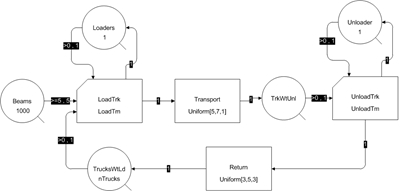### Multi-Step Activities in EZStrobe – Identical Consecutive Steps

Sometimes the modeler has the choice, or the data, to model an activity that consists of n multiple identical steps as one long activity or as a n consecutive shorter activities. An example is loading a flatbed truck with five pre-fabricated beams using a crane. The modeler can choose to represent the loading activity as one activity that represents loading all five beams, or as five consecutive activities each of which represents loading one beam.

The most effective way to model such cases is to use one single activity that captures all the steps. This is possible if:

1. The resources involved remain committed to the activity during all steps (e.g.. if a crane starts loading a flatbed only when five beams are available and it never stops to do something else until all five beams are loaded.
2. The distribution of the time required for the completion of all the steps required is available (e.g., the modeler knows the distribution of the time it takes to load five beams).

The EZStrobe network below (click it to see in detail) illustrates two such activities: One for loading five beams at the fabrication site and another for unloading them at the job site:The time to load (LoadTm) or unload (UnloadTm) all five beams is defined using an EZStrobe Result that contains the appropriate distribution, for example ScaledBeta[5,10,8,8] (minutes). Note that EZStrobe Results must be used in order for each activity instance to sample a new duration to obtain its time. If EZStrobe Parameters are used instead, EZStrobe will sample once at the start of the simulation to establish the value of each parameter, which will retain that value from then on. As a consequence, all instances of each activity will have the same duration rather than be sampled from the distribution to establish the time of each instance.

The exact same network can be used when the distribution of the time required for the completion of all the steps required is not available, if:

1. The distribution of each step is known (e.g., the modeler knows the distribution of the time it takes to load one beam).
2. The number of multiple identical steps that make up a long activity is relatively small.

This can be achieved by setting the distribution of the time for the activity as the explicit sum of samples from the distribution of the time for each step.  For example, if the modeler knows that the distribution of the time to load or unload one beam is Uniform[1,2] (minutes), the LoadTm and UnloadTm EZStrobe Results can be defined as Uniform[1,2]+Uniform[1,2]+Uniform[1,2]+Uniform[1,2]+Uniform[1,2]. If the number of steps is large then it becomes cumbersome to define the results appropriately, although EZStrobe will actually allow the formula for a result to be up to 64k characters in length.

Note that the sum of 5 independent samples from Uniform[1,2] is not the same as 5*Uniform[1,2], which multiplies one sample by 5 and is uniformly distributed between 5 and 10. The sum of 5 independent samples from a uniform distribution between 1 and 2 is actually an Irwin-Hall distribution with parameter 5 (quite close to a Beta distribution with both shape parameters set to 8), scaled and translated to the range [5,10].

Please see the article Multi-Step Activities in EZStrobe – Non Identical Consecutive Steps, to see how to model multi-step activities when the steps required are not Independent and Identically Distributed (IID), when the number of steps required is variable, or when the multi-step activity can be interrupted between steps.

The model for this article can be obtained here. It has a seed and uses multiple random number streams so that it yields the exact same output as other models that are supposed to be identical.

EZStrobe is based upon work supported by the National Science Foundation under Grant No. 9733267. Any opinions, findings, and conclusions or recommendations are those of the author(s) and do not necessarily reflect the views of the National Science Foundation.